Prime Numbers / L.C.M. / Zeta Functions HomePage

# An Approach to Prime Numbers , the L.C.M. , and the Zeta Functions

## Summary & Key Findings

• ### Finding #1 - the L.C.M. as an indicator :

• Take the 30 consecutive { natural numbers } from [ 2 ] to [ 31 ] :

• If all 30 numbers are { prime numbers } , the L.C.M. would have been [ 31-Factorial ] ;

• If the { prime factors } of the 30 numbers comes-down to one single { prime number } , the L.C.M. would have been [ 31 ] ;

• [ 31 ] being the largest number of the lot .

The fact the L.C.M. is somewhere in-between serves as a first-indicator as to how many { prime numbers } there are .

• ### Finding #2 - the lower-bound estimate of [ pi-of-(M) ] via the L.C.M. :

• We set up the L.C.M.-based function { Lamda-of-(M) } :

•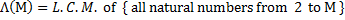And we were able to establish :

•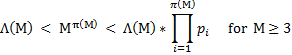where [ pi-of-(M) ] is the { prime number counting function } .

The term on-the-very-right under the [ product sign ] is then the [ product-of-primes ] up to [ M ] .

• ### Finding #3 - A Fast-Track Approach to identifying { prime numbers } :

• [ 2 x 3 ] = 6 , so that [ 1 mod 6 ] and [ 5 mod 6 ] are candidates for { prime numbers } next .

We then use the { product-of-primes } series as the [ modulo's ] series to further identify { prime numbers } , i.e. the series :

• [ modulo 6 / 30 / 210 / 2310 / 30030 / 510510 / 9699690 / etc. ] ;

with some success initially .

• ### Finding #4 - Proposing an Inequality in lieu of the Euler Product Formula :

• In lieu of the Euler Product Formula for { real values of [ s ] } :

•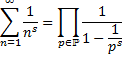we propose the { Fung Inequality } , as follows :

•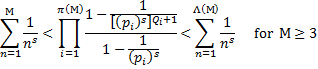• ### Finding #5 - Step Functions that pops at { primes } and { power-of-primes } :

• Riemann's {prime counting function } is the [ F(x) ] function and he used its derived function [ f(x) ] :

•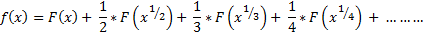as the core function in his investigations of { prime numbers } .

The f(x) fucntion is a { step function } that [ pops ] whenever the value of [ x ] hits a { prime } or a { power-of-prime } .

• So does our [ Lamda-of-(M) ] function .